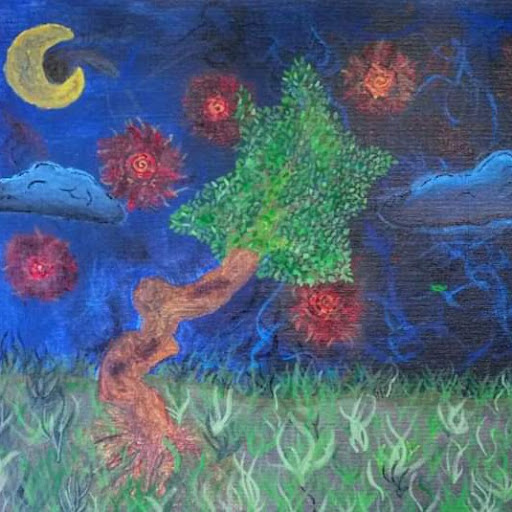# Is there a way to widen the GUI box in python?

I am trying to make a simple sentence generator. The code works but I just want to know if there are any ways to expand the GUI entry box?

This is how it looks like:Any help would be appreciated.

``````import tkinter as tk
from tkinter import Entry
import random
window = tk.Tk()

def movingVerb():
verbs = ["goes to", "walks through", "runs through"]
moveVerb = random.choice(verbs)
return moveVerb
def anObject():
anObject = objectEntry.get()
def Generate():
moveVerb = movingVerb()
place = placeEntry.get()
noun = objectEntry.get()
sentence = UserName + " " + moveVerb + " a " + place + " and finds a " + noun + " ."
result.delete(0, tk.END)
result.insert(0, sentence)

UserNameLabel = tk.Label(window, text="Enter a name: ")
nameEntry = tk.Entry(window)
anObjectLabel = tk.Label(window,text="Enter an object: ")
objectEntry = tk.Entry(window)
placeLabel = tk.Label(window, text="Enter a place: ")
placeEntry = tk.Entry(window)
button = tk.Button(window, text="Generate story", command=Generate)
result = tk.Entry(window)

nameEntry.pack()
anObjectLabel.pack()
objectEntry.pack()
placeLabel.pack()
placeEntry.pack()
button.pack()
result.pack()
window.mainloop()

``````On Best Solutions

use place instead of pack, and you can do this sweet method here.

`````` placeLabel.place(relwidth=0.25, relheight=0.90)
``````On

If you want to expand widgets when you resize window then you can use `expand` and `fill` in `pack()` in different combinations. All depends on which element you want to expand and in which direction.

``````.pack(fill='x')
.pack(fill='y')
.pack(fill='both')
.pack(expand=True, fill='x')
.pack(expand=True, fill='y')
.pack(expand=True, fill='both')
``````If you want to resize window to see all new text in `Entry` then you can use `widget.configure(width=...)`. Entry uses number of chars as width.

You can use lenght of sentence to change width

``````result.configure(width=len(sentence))
``````

or to keep width at least 20 chars

``````length = max(20, len(sentence))
result.configure(width=length)
``````

Full code

``````import tkinter as tk
import random

# --- functions ---

verbs = ["goes to", "walks through", "runs through"]

def generate():
user_name = name_entry.get()
move_verb = random.choice(verbs)
place = place_entry.get()
noun = object_entry.get()
sentence = '{} {} a {} and finds a {}.'.format(user_name, move_verb, place, noun)
result.delete(0, 'end')
result.insert(0, sentence)
length = max(20, len(sentence))
result.configure(width=length)

# --- main ---

window = tk.Tk()

user_name_label = tk.Label(window, text="Enter a name: ")
name_entry = tk.Entry(window)
object_label = tk.Label(window,text="Enter an object: ")
object_entry = tk.Entry(window)
place_label = tk.Label(window, text="Enter a place: ")
place_entry = tk.Entry(window)
button = tk.Button(window, text="Generate story", command=generate)
result = tk.Entry(window)

user_name_label.pack(expand=True, fill='both')
name_entry.pack(fill='both')
object_label.pack(expand=True, fill='both')
object_entry.pack(fill='both')
place_label.pack(expand=True, fill='both')
place_entry.pack(fill='both')
button.pack(expand=True, fill='both')
result.pack(fill='both')

window.mainloop()
``````